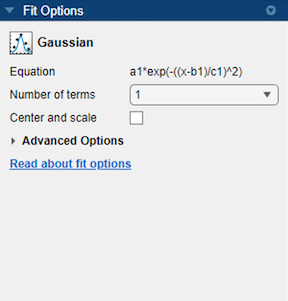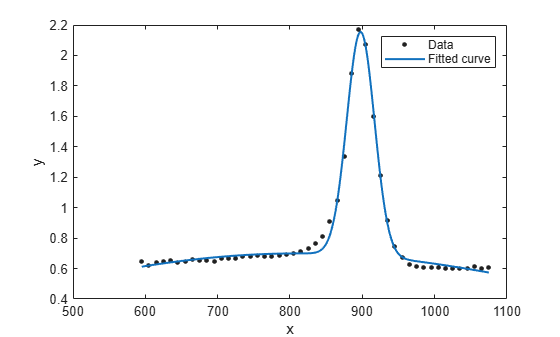Gaussian Models

The Gaussian model fits peaks, and is given by

$y=\sum _{i=1}^{n}{a}_{i}{e}^{\left[-{\left(\frac{x-{b}_{i}}{{c}_{i}}\right)}^{2}\right]}$

where a is the amplitude, b is the centroid (location), c is related to the peak width, n is the number of peaks to fit, and 1 ≤ n ≤ 8.

Gaussian peaks are encountered in many areas of science and engineering. For example, Gaussian peaks can describe line emission spectra and chemical concentration assays.

Fit Gaussian Models Interactively

1. Open the Curve Fitting app by entering cftool. Alternatively, click Curve Fitting on the Apps tab.

2. In the Curve Fitting app, select curve data (X data and Y data, or just Y data against index).

Curve Fitting app creates the default curve fit, Polynomial.

3. Change the model type from Polynomial to Gaussian.You can specify the following options:

• Choose the number of terms: 1 to 8.

Look in the Results pane to see the model terms, the values of the coefficients, and the goodness-of-fit statistics.

• (Optional) Click Fit Options to specify coefficient starting values and constraint bounds, or change algorithm settings.

The toolbox calculates optimized start points for Gaussian models, based on the current data set. You can override the start points and specify your own values in the Fit Options dialog box.

Gaussians have the width parameter c1 constrained with a lower bound of 0. The default lower bounds for most library models are -Inf, which indicates that the coefficients are unconstrained.

For more information on the settings, see Specifying Fit Options and Optimized Starting Points.

Fit Gaussian Models Using the fit Function

This example shows how to use the fit function to fit a Gaussian model to data.

The Gaussian library model is an input argument to the fit and fittype functions. Specify the model type gauss followed by the number of terms, e.g., 'gauss1' through 'gauss8' .

Fit a Two-Term Gaussian Model

Load some data and fit a two-term Gaussian model.

[x,y] = titanium;
f = fit(x.',y.','gauss2')
f =
General model Gauss2:
f(x) =  a1*exp(-((x-b1)/c1)^2) + a2*exp(-((x-b2)/c2)^2)
Coefficients (with 95% confidence bounds):
a1 =        1.47  (1.426, 1.515)
b1 =       897.7  (897, 898.3)
c1 =       27.08  (26.08, 28.08)
a2 =      0.6994  (0.6821, 0.7167)
b2 =       810.8  (790, 831.7)
c2 =       592.9  (500.1, 685.7)
plot(f,x,y)